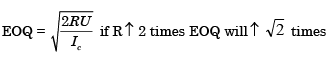Courses

# CPM, PERT & Inventory Control - MCQ Test 2

## 30 Questions MCQ Test RRB JE for Mechanical Engineering | CPM, PERT & Inventory Control - MCQ Test 2

Description
This mock test of CPM, PERT & Inventory Control - MCQ Test 2 for Mechanical Engineering helps you for every Mechanical Engineering entrance exam. This contains 30 Multiple Choice Questions for Mechanical Engineering CPM, PERT & Inventory Control - MCQ Test 2 (mcq) to study with solutions a complete question bank. The solved questions answers in this CPM, PERT & Inventory Control - MCQ Test 2 quiz give you a good mix of easy questions and tough questions. Mechanical Engineering students definitely take this CPM, PERT & Inventory Control - MCQ Test 2 exercise for a better result in the exam. You can find other CPM, PERT & Inventory Control - MCQ Test 2 extra questions, long questions & short questions for Mechanical Engineering on EduRev as well by searching above.
QUESTION: 1

### The project activities, precedence relationships and durations are described in the table. The critical path of the project is: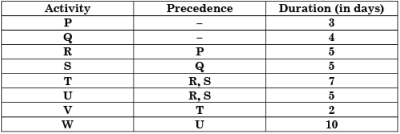Solution:

Q – S – V – W is haring maximum duration = 24 days so it is the critical path.

QUESTION: 2

### In a machine shop, pins of 15 mm diameter are produced at a rate of 1000 per month and the same is consumed at a rate of 500 per month. The production and consumption continue simultaneously till the maximum inventory is reached. Then inventory is allowed to reduce to zero due to consumption. The lot size of production is 1000. If backlog is not allowed, the maximum inventory level is:

Solution:

MAXIMUM INVENTORY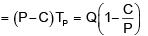QUESTION: 3

### A project has six activities (A to F) with respective activity durations 7, 5, 6, 6, 8, 4 days. The network has three paths A-B, C-D and E-F. All the activities can be crashed with the same crash cost per day. The number of activities that need to be crashed to reduce the project duration by 1 day is:

Solution:
QUESTION: 4

Consider the following data for an item.

Annual demand: 2500  units per year Ordering cost: Rs. 100 per order Inventory holding rate: 25% of unit price.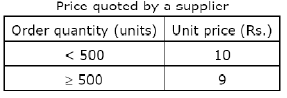The optimum order quantity ?

Solution:

D = 2500 units/years
Co = Rs. 100/order
Ch = (0.25) Unit + Price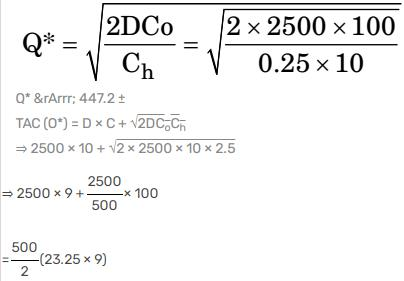⇒23562.5
Since Total cost minimum at Q = 500 units
∴ the optimal order quality is Q = 500 units

QUESTION: 5

Consider the following statements:

PERT considers the following time estimates
1. Optimistic time
2. Pessimistic time
3. Most likely time

Which of the statements given above are correct?

Solution:
QUESTION: 6

In computing Wilson's economic lot size for an item, by mistake the demand rate estimate used was 40% higher than the tree demand rate. Due to this error in the lot size computation, the total cost of setups plus inventory holding per unit time. Would rise above the true optimum by approximately

Solution: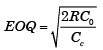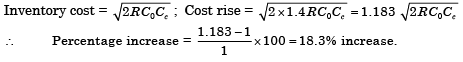QUESTION: 7

Consider the following statements with respect to PERT

1. It consists of activities with uncertain time phases
2. This is evolved from Gantt chart
3. Total slack along the critical path is not zero
4. There can be more than one critical path in PERT network
5. It is similar to electrical network

Which of the statements given above are correct?

Solution:
QUESTION: 8

One of the following statements about PRS (Periodic Reordering System) is not true. Identify

Solution:
QUESTION: 9

Dummy activities are used in a network to:

Solution:
QUESTION: 10

The net requirements of an item over 5 consecutive weeks are 50-015-20-20. The inventory carrying costs are Re. 1 per item per week and Rs. 100 per order respectively. Starting inventory is zero. Use “Least Unit Const Technique” for developing the plan. The const of the plan (in Rs.) is:

Solution:
QUESTION: 11

A PERT activity has an optimistic time estimate of 3 days, a pessimistic time estimate of 8 days, and a most likely time estimate of 10 days. What is the expected time of this activity?

Solution: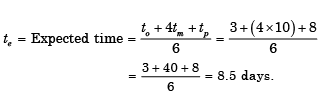QUESTION: 12

Which of the following are the benefits of inventory control?

1. Improvement in customers relationship.
2. Economy in purchasing.
3. Elimination of the possibility of duplicate ordering.

Select the correct answer using the code given below:

Solution:
QUESTION: 13

Which one of the following statements is not correct?

Solution:

(a) PERT → Event oriented
CPM → Activity oriented
(b) PERT → 3 tim e esti mates are made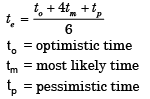CPM → on ly one ti me estimate
(c) In PERT slack is calculated, CPM floats calculated
(d) Both PERT and CPM are used for project situation.

QUESTION: 14

In ABC analysis, A items require:

Solution:
QUESTION: 15

If the earliest starting time for an activity is 8 weeks, the latest finish time is 37 weeks and the duration time of the activity is 11 weeks, then the total float is equal to:

Solution:

T.F. = LSi− ESij − tij = 37 − 8 − 11 = 18

QUESTION: 16

Classifying items in A, B and C categories for selective control in inventory management is done by arranging items in the decreasing order of:

Solution:
QUESTION: 17

The earliest occurrence time for event '1' is 8 weeks and the latest occurrence time for event' I' is 26 weeks. The earliest occurrence time for event '2' is 32 weeks and the latest occurrence time for event '2' is 37 weeks. If the activity time is 11 weeks, then the total float will be:

Solution:

Total float = 37 – 8 – 11 = 18 days.

QUESTION: 18

Assertion (A): Selective control manages time more effectively.

Reason (R): ABC analysis is based on Pareto distribution.

Solution:
QUESTION: 19

Which of the following are the guidelines for the construction of a network diagram?

1. Each activity is represented by one and only one arrow in the network.
2. Two activities can be identified by the same beginning and end events.
3. Dangling must be avoided in a network diagram.
4. Dummy activity consumes no time or resource.

Select the correct answer using the codes given below:

Solution:
QUESTION: 20

ABC analysis in materials management is a method of classifying the inventories based on

Solution:
QUESTION: 21

Earliest finish time can be regarded as

Solution:
QUESTION: 22

Consider the following statements:

1. ABC analysis is based on Pareto's principle
2. FIFO and LIFO policies can be used for material valuation in materials management.
3. Simulation can be Used for inventory control.
4. EOQ (Economic Order Quantity) formula ignores variations in demand pattern.

Of these statem ents:

Solution:
QUESTION: 23

Consider an activity having a duration time of Tij. E is the earliest occurrence time and L the latest occurrence time (see figure given).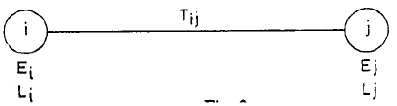Consider the following statements in this regard:

1. Total float = Lj - Ei - Tij
2. Free float = Ej - Ei - Tij
3. Slack of the tail event = Lj- Ei

Of these statem ents:

Solution:

QUESTION: 24

Out of the following item listed below, which two items you would consider under category (c) under ABC analysis: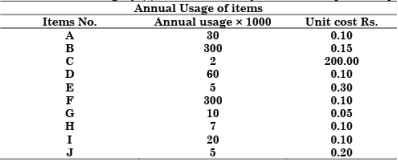Solution:
QUESTION: 25

What is the additional time available for the performance of an activity in PERT and CPM calculated on the basis that all activities will start at their earliest start time, called?

Solution:
QUESTION: 26

In the ABC method of inventory control, Group A constitutes costly items. What is the usual percentage of such items of the total items?

Solution:
QUESTION: 27

Which one of the following networks is correctly drawn?

Solution:

Diagram (a) is correct as in (b) & (c) diagrams backward arrows are seen which is not correct. In (d) both activity is dummy it is also not correct.

QUESTION: 28

Which one of the following is correct?
In the basic EOQ model, if lead time increases from 5 to 10 days, the EOQ will:

Solution:
QUESTION: 29

The essential condition for the decompression of an activity is:

Solution:
QUESTION: 30

In the EOQ model, if the unit ordering cost is doubled, the EOQ

Solution: